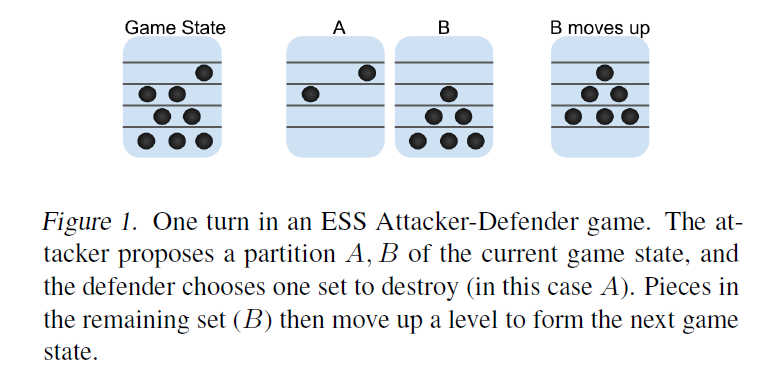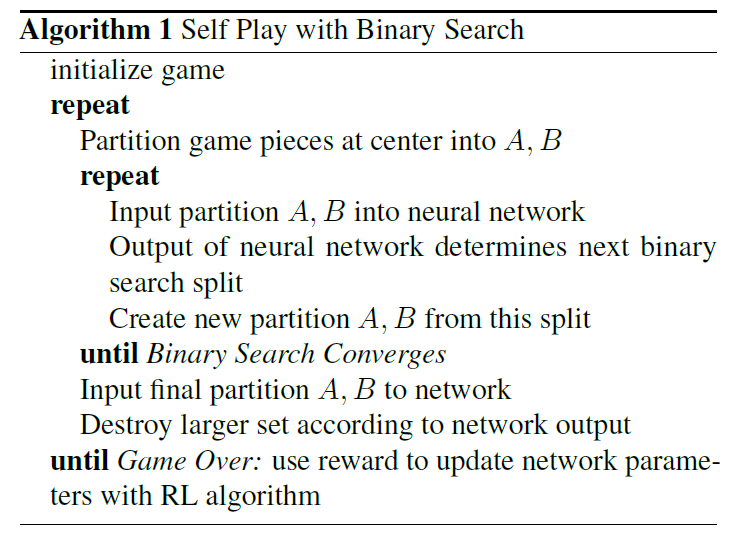# 多智能体强化学习算法

## Can Deep Reinforcement Learning solve Erdos-Selfridge-Spencer Games?

Posted by MY on August 6, 2020

## 一、问题attacker-defender game包含两个玩家：移动碎片的攻击者和破坏碎片的防御者。比如，游戏具有一组从0到K层的楼层，并且在这些楼层上初始化了N个碎片。 攻击者的目标是至少使他们的碎片之一达到K层，而防御者的目标是在这发生之前销毁所有N枚碎片。在每个回合中，攻击者都会选择一个现存碎片的划分：$A$和$B$。 防守者选择其中一组进行破坏并从游戏中移除。另一组中的所有碎片均上移一层。 当一个或多个碎片达到楼层K，或者所有碎片都被破坏时，游戏结束。

## 二、解法

• Potential Function：给定游戏状态$S=\left(n_{0}, n_{1}, \dots, n_{K}\right)$，$n_{i}$为在$i$层的碎片数，该函数定义为$\phi(S)=\sum_{i=0}^{K} n_{i} 2^{-(K-i)}$。
• 考虑一个具有K层和N个碎片的游戏，该碎片放置在不同楼层上的任意位置，初始状态为$S_{0}$。如果$\phi(S_{0}) < 1$，防守者必胜；如果$\phi(S_{0}) > 1$，进攻者必胜。
• $\phi(S_{0}) < 1$时，防守者最优策略是摧毁$A$和$B$中Potential值更大的一组；$\phi(S_{0}) > 1$时，进攻者最优策略是划分两组Potential值$\ge 0.5$的$A$和$B$。但由于这种最优解需要探索的动作空间太大，本文提出了一种新的最优方法，即在这种设置下，存在$A$和$B$使得$A$包含的碎片所属楼层全部大于某值$l$，$B$包含的碎片所属楼层全部小于某值$l$。因此攻击者只要探索选取哪个楼层进行划分$A$和$B$即可。

## 三、实验内容## 五、优点

##### Share